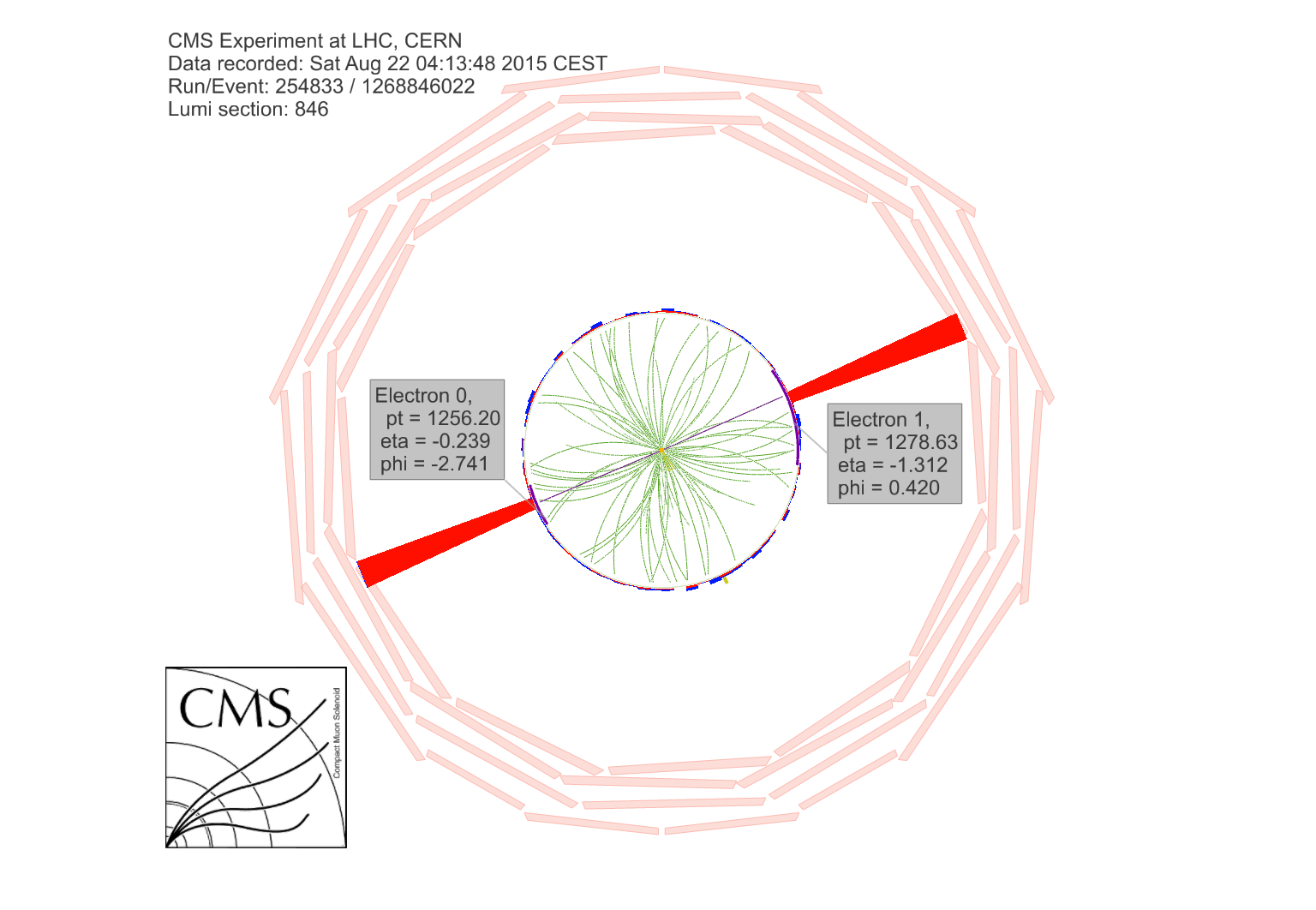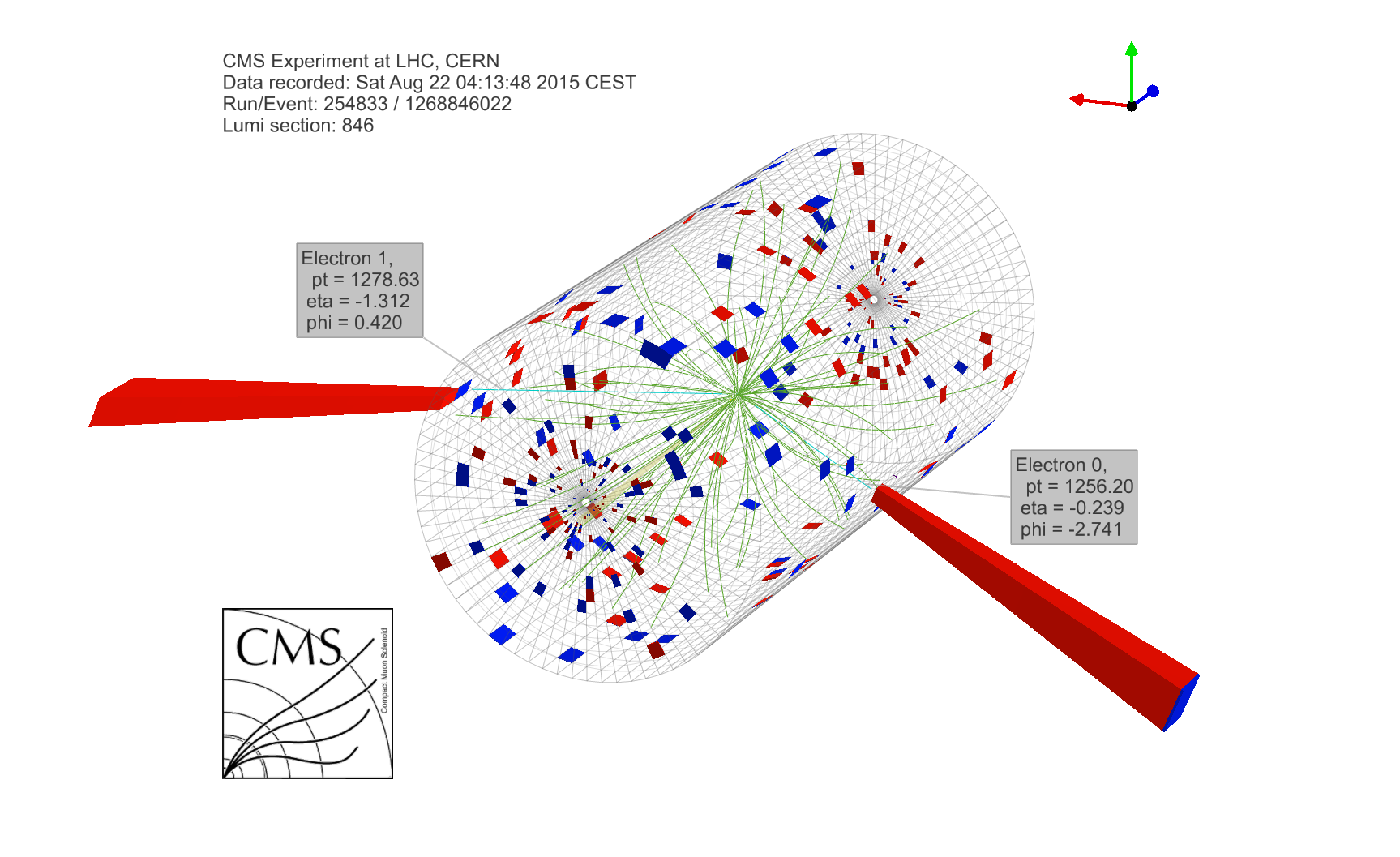## Thursday, September 03, 2015

### CMS: a $$2.9\TeV$$ electron-positron pair resonance

Bonus: An ATLAS $$\mu\mu j$$ event with $$m=2.9\TeV$$ will be discussed at the end of this blog post.
A model with exactly this prediction was published in June

Two days ago, I discussed four LHC collisions suggesting a particle of mass $$5.2\TeV$$. Today, just two days later, Tommaso Dorigo described a spectacular dielectron event seen by CMS on August 22nd. See also the CERN document server; CERN graduate students have to prepare a PDF file for each of the several quadrillion collisions. ;-)

On that Tuesday, the world stock markets were just recovering from the two previous cataclysmic days while the CMS detector enjoyed a more pleasing day with one of the $$13\TeV$$ collisions that have turned the LHC into a rather new kind of a toy.This is how the outcome of the collision looked from the direction of the beam. The electron and positron were flying almost exactly in the opposite direction, each having about $$1.25\TeV$$ of transverse energy. A perfectly balanced picture.

You may see the collision from another angle, too:The electron-positron pair is the only notable thing that is going on.

The fun is that no such high-energy collision has been seen at the $$8\TeV$$ run – even though it has performed more than 100 times greater a number of collisions than the ongoing $$13\TeV$$ run in 2015. When you demand truly highly energetic particles in the final state, the weakness of the $$8\TeV$$ run in 2012 becomes self-evident.

The expected number of similar collisions with the invariant mass$M_{e^+e^-}\gt 2.5\TeV$ seen in the CMS dataset of 2015 (so far) has been estimated as $$\langle N \rangle =0.002$$. Clearly, this number – because it is so small that we may neglect the possibility that more than 1 such event arises – may be interpreted as the probability that one event (and not zero events) take place. For the mass above $$2.85\TeV$$, you would almost certainly get a probability $$0.001$$ or less.

If you take the estimate $$p=0.002$$ seriously, it means that either the CMS detector has been 1:500 "lucky" to see a high-energy event that is actually noise; or it is seeing a new particle that may decay to the electron-positron pair.

Such a new particle would probably be neutral from all points of view. It could be a heavier cousin of the $$Z$$-boson, a $$Z'$$-boson. That would be the gauge boson associated with a new $$U(1)_{\rm new}$$ gauge symmetry. Most types of vacua in string theory tend to predict lots of these additional $$U(1)$$ groups.

And your humble correspondent can even offer you a paper that predicts a $$Z'$$-boson of mass $$2.9\TeV$$. See the bottom of page 10 here. (Sadly, they made the prediction less accurate in v2 of their preprint.) The left-right-symmetric model in the paper also intends to explain the excesses near $$2\TeV$$ – as a $$W'$$-boson. The model is lepto-phobic (LP) which means that only right-handed quarks are arranged to doublets of $$SU(2)_R$$ while the right-handed leptons remain $$SU(2)_R$$ singlets. It's the model with the Higgs triplet (LPT) that gives the right $$Z'$$-boson mass.

Just for fun, let me show you the calculation of the invariant mass. The coordinates of the two electron-like particles are written as$\eq{ p_T &= 1.27863\TeV\\ \eta &= - 1.312\\ \phi &= 0.420 }$ and $\eq{ p_T &= 1.25620\TeV\\ \eta &= - 0.239\\ \phi &= -2.741 }$ One may convert these coordinates to the Cartesian coordinates$\eq{ p_x &= p_T\cos \phi\\ p_y &= p_T\sin \phi\\ p_z &= p_T \sinh \eta \\ E &= p_T \cosh \eta }$ in the approximation $$m_e\ll E$$ i.e. $$m_e\sim 0$$: feel free to check that the 4-vector above is identically light-like. The two 4-vectors (in the order I chose above) are therefore$\eq{ \frac{p_A^\mu }{ {\rm TeV}}&= (1.16750, 0.521375, -2.20200, 2.54631) \\ \frac{p_B^\mu }{ {\rm TeV}}&= (-1.15675, -0.48987, -0.30310, 1.29225) }$ where the last coordinate is the energy. Now, because these 4-vectors are null, $(p_A^\mu+p_B^\mu)^2 = 2p_A^\mu p_{B,\mu} = (2.908\TeV)^2$ in the West Coast metric convention. You're invited to check it. Thanks to the Higgs Kaggle contest, I gained some intuition for the $$(p_T,\eta,\phi)$$ coordinates. ;-)

In a few more weeks, we should see whether this highly energetic electron-positron event was a fluke or something much more interesting... You know, the progress on the energy frontier has been rather substantial. Note that $$13/8=1.625$$, an increase by 62.5%.

Lots of particles – the $$W$$-bosons, the $$Z$$-boson, the Higgs boson, and the top quark – are confined in the interval $$70\GeV,210\GeV$$ – safely four types of particles in an interval whose upper bound is thrice the lower bound. Now, we can produce particles with masses up to $$5\TeV$$ or so. Why shouldn't we find any new particles with masses between $$175\GeV$$ and $$4,900\GeV$$ – an interval whose ratio of limiting energies is twenty-eight?

It's quite some jump, isn't it? ;-) It could harbor lots of so far secret and elusive animals.

Next Monday, the full-fledged physics collisions should resume and continue through the early November.